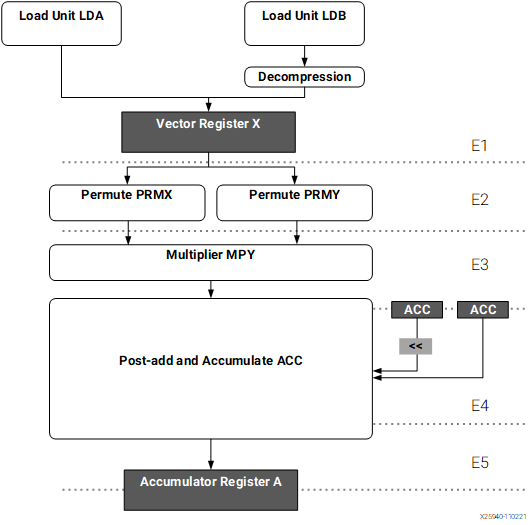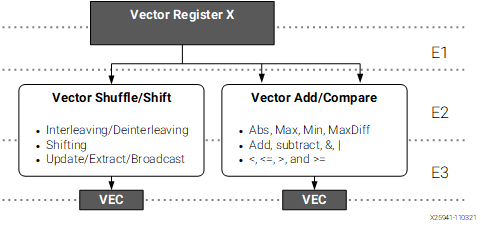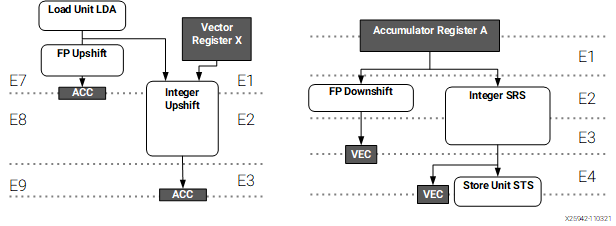# Fixed-Point Vector Unit

Document ID
AM020
Release Date
2023-11-10
Revision
1.2 English

## AIE-ML Fixed-Point Vector Unit

The following is a block diagram of the fixed-point vector data path. The datapath is split into five pipeline stages.

Figure 1. Pipeline Diagram of AIE-ML Fixed-point Vector Unit Multiplication PathsThe features of the units in the datapath are as follow:

• The multiplier unit is fed by the output of the permute blocks. The vector adder is in a separate functional unit together with a vector shuffle and shift datapath.
• There are two permute units PRMX and PRMY that handle a set of permutes of X vector registers.
• In addition to the permute and multiplier, there are two additional vector units: shuffle/shift and add/compare. The input comes directly from two vector registers and the results are stored back in the vector registers. The supported bit-width modes are (both signed and unsigned):
• 16 lanes of 32-bit
• 32 lanes of 16-bit
• 64 lanes of 8-bit
The unit supports lane-by-lane control whether addition or subtraction is performed.
Figure 2. Pipeline Diagram of AIE-ML Fixed-point Vector Unit Shuffle/Shift and Adder PathsThe previous image shows that in addition to the vector adder, there is a vector shuffle and shift datapath. The vector shift unit takes one or two 512-bit vector registers as an input and produces one 512-bit output vector. It supports the following modes:

• Standard right shift with 8-bit granularity
• Shift and push in scalar value either at the left or right-hand side. An 8, 16, or 32-bit lane can be shifted into the LSB lane of a 512-bit vector register, and all existing values are shifted one lane up. The value of MSB lane is dropped.

The shuffle unit allows different modes to transform the input vectors. It supports the following features:

• Interleaving and deinterleaving of values at 8-bit, 16-bit, and 32-bit
• Extraction of upper and lower half of the transformed input.

## Fixed-Point SRS and UPS Conversions

A block diagram of the units is shown in the following figure.

Figure 3. Fixed-point SRS and UPS DatapathThe SRS unit reads an accumulator register, performs the conversion, and restores the result either back to the vector register or directly to memory. The UPS unit reads a vector register directly from memory or a register and stores the result into an accumulator register. The supported modes include:

• 32 lanes of 8-bit to/from 32-bit conversion
• 32 lanes of 16-bit to/from 32-bit conversion
• 16 lanes of 16-bit to/from 64-bit conversion
• 16 lanes of 32-bit to/from 64-bit conversion

A floating-point conversion mode is also supported. It converts bfloat16 to single precision or vice versa. The modes supported are:

• 16 lanes of fp32 accumulators to bfloat16 vector registers
• 16 lanes of bfloat16 vector registers to fp32 accumulators

In addition (not shown in the figure), the unit also supports floating-point to integer conversion: 16 lanes of bfloat16 vector registers to 32-bit signed registers.